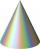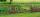Cone and the ratio

Rotational cone has a height 23 cm and the ratio of the base surface to lateral surface is 7: 9. Calculate a surface of the base and the lateral surface.

Result

S1 =  2544.79 cm2
S2 =  3271.87 cm2

Solution:Leave us a comment of example and its solution (i.e. if it is still somewhat unclear...):Be the first to comment!To solve this verbal math problem are needed these knowledge from mathematics:

Pythagorean theorem is the base for the right triangle calculator.

Next similar examples:

1. Area of the coneCalculate the surface area of the cone, you know the base diameter 25 cm and a height 40 cm.
2. Cone 15The radius of the base of a right circular cone is 14 inches and it's height 18 inches. What is the slant height?
3. Pile of sandA large pile of sand has been dumped into a conical pile in a warehouse. The slant height of the pile is 20 feet. The diameter of the base of the sand pile is 31 feet. Find the volume of the pile of sand.
4. Truncated cone 5The height of a cone 7 cm and the length of side is 10 cm and the lower radius is 3cm. What could the possible answer for the upper radius of truncated cone?
5. Two gardenersThe garden with an area of 81 square meters was divided by two gardeners in a ratio of 4: 5. How much did the second gardener get more first?
6. Broken treeThe tree was 35 meters high. The tree broke at a height of 10 m above the ground. Top but does not fall off it refuted on the ground. How far from the base of the tree lay its peak?
7. Trapezium basesFind the trapezium height if a = 8 cm and c = 4 cm if its content 21 square centimeters.
8. Four ropesTV transmitter is anchored at a height of 44 meters by four ropes. Each rope is attached at a distance of 55 meters from the heel of the TV transmitter. Calculate how many meters of rope were used in the construction of the transmitter. At each attachment.
9. WindbreakA tree at a height of 3 meters broke in the windbreak. Its peak fell 4.5 m from the tree. How tall was the tree?
10. Chord circleThe circle to the (S, r = 8 cm) are different points A, B connected segment /AB/ = 12 cm. AB mark the middle of S'. Calculate |SS'|. Make the sketch.
11. HypotenuseCalculate the length of the hypotenuse of a right triangle if the length of one leg is 4 cm and its content area is 16 square centimeters.
12. Rectangular triangle PQRIn the rectangular triangle PQR, the PQ leg is divided by the X point into two segments of which longer is 25cm long. The second leg PR has a length 16 cm. The length of the RX is 20 cm. Calculate the length p of side RQ. The result is round to 2 decimal
13. EmbankmentPerpendicular cross-section of the embankment around the lake has the shape of an isosceles trapezoid. Calculate the perpendicular cross-section, where bank is 4 m high the upper width is 7 m and the legs are 10 m long.
14. Equilateral triangleThe equilateral triangle has a 23 cm long side. Calculate its content area.
15. Simplify 2Simplify expression: 5ab-7+3ba-9
16. Holidays - on poolChildren's tickets to the swimming pool stands x € for an adult is € 2 more expensive. There was m children in the swimming pool and adults three times less. How many euros make treasurer for pool entry?
17. AcreageWhat acreage has a rectangular plot whose diagonal is 34 meters long and one side has a length of 16 meters. ?